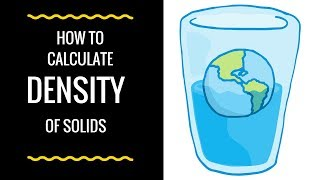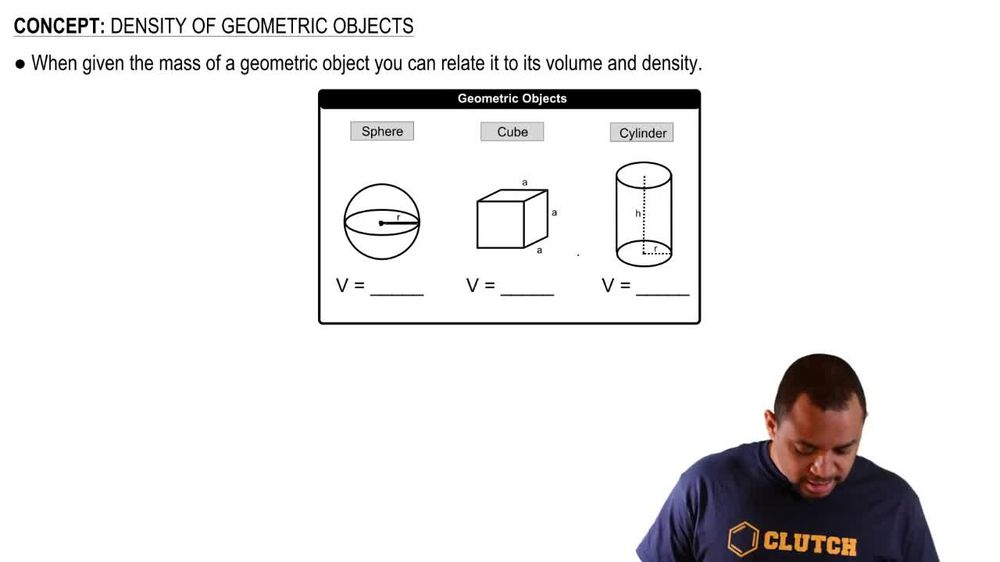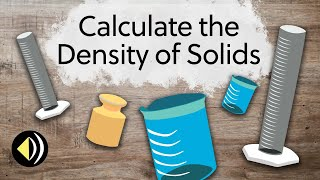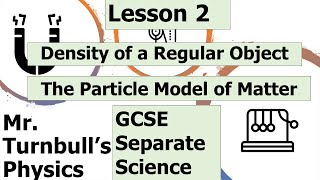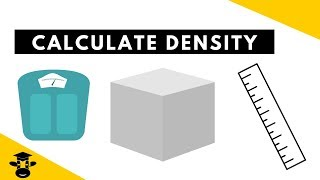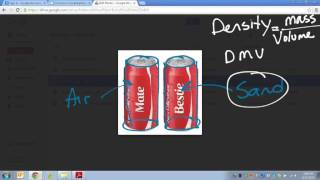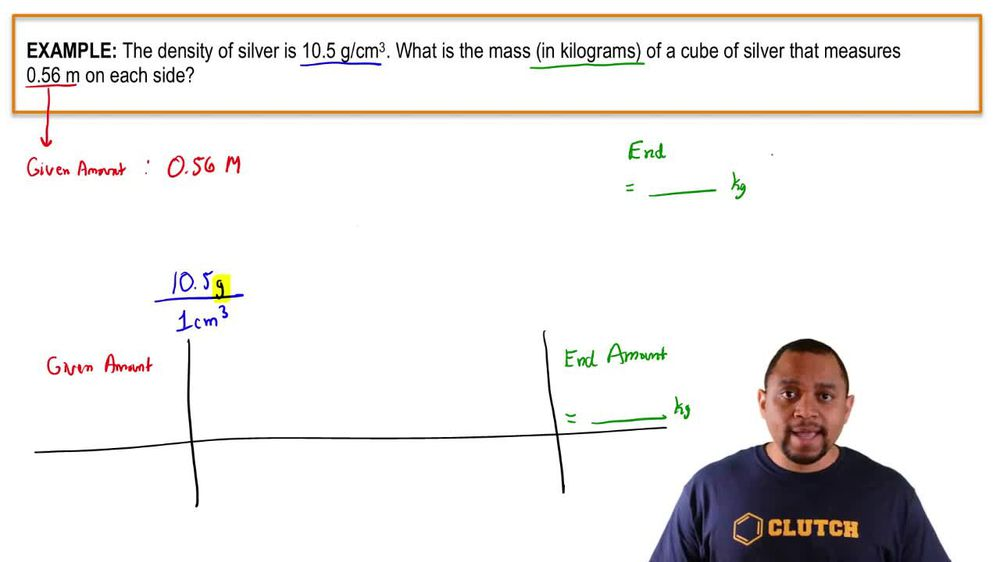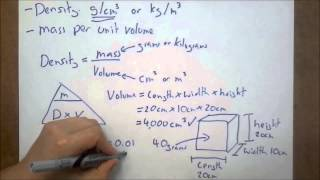Start typing, then use the up and down arrows to select an option from the list.
1. 1. Intro to General Chemistry2. Density of Geometric Objects
Problem

# Lead metal can be extracted from a mineral called galena, which contains 86.6% lead by mass. A particular ore contains 68.5% galena by mass. If the lead can be extracted with 92.5% efficiency, what mass of ore is required to make a lead sphere with a 5.00-cm radius?

Relevant Solution3m
Play a video:
Hi everyone today. We have a question telling us the full loring is an al trope of carbon whose molecule consists of carbon atoms connected by single and double bonds. So as to perform a closed structure With few strings of 5 - seven atoms. Buckminster fuel ring is a type of food coloring that has a density of 1.65 g per cm cubed. The diameter of one molecule is 1.1 nanometers. And our goal is to calculate the mass of one molecule of Buckminster fuller ring. So we need to remember that density equals mass divided by volume and the volume of a sphere equals four thirds times pi times are radius cube. So we're going to take this and plug it in for our volume here which gives us density equals mass Divided by 4/3. Hi times are radius cube. Now our diameter is 1. nmeter To get our radius from that. We simply divide by two which equals 0.55 nm. And we need that in centimeters. So we're going to take our 0.55 nanometers and multiply by one m over 10 to the ninth nanometers times 10 squared centimeters over one m. And our nanometers are going to cancel out and our meters are going to cancel out. And that's going to give us 5.5 Times 10 to the negative eight cm. So now we can plug that in. So mass is going to equal density times four thirds pie are cute and mass Will equal the 1.65 grams per centimeter cubed. Given to us in the prompt times four thirds pie times 5.5 Times to the negative eight cm Q, And that equals 1.15 times to the -21 g. And that is our final answer. Thank you for watching. Bye.